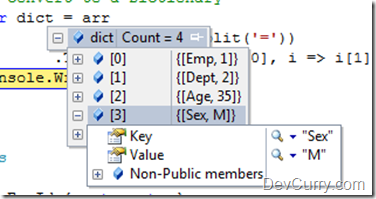# Convert String to Dictionary using LINQ

 Tweet

We were working on a requirement where we had a string containing key-value pairs as shown below:

`string htmlStr = "[Emp=1][Dept=2][Age=35][Sex=M]";`

We had to convert this string to a Dictionary in the simplest possible way. Here’s how it can be done using Enumerable.ToDictionary()

C#

`public static void Main(){    string htmlStr = "[Emp=1][Dept=2][Age=35][Sex=M]";    string[] arr = htmlStr.Split(new[] { '[', ']' },        StringSplitOptions.RemoveEmptyEntries);    // convert to a Dictionary    var dict = arr            .Select(x => x.Split('='))            .ToDictionary(i => i, i => i);         }`

VB.NET (Converted Code)

`Public Shared Sub Main()    Dim htmlStr As String = "[Emp=1][Dept=2][Age=35][Sex=M]"    Dim arr() As String = htmlStr.Split( { "["c, "]"c }, StringSplitOptions.RemoveEmptyEntries)    ' convert to a Dictionary    Dim dict = arr.Select(Function(x) x.Split("="c)).ToDictionary(Function(i) i(0), Function(i) i(1))End Sub`

As you can see, we first split the string and then use the ToDictionary method to convert the split string into a Dictionary object

OUTPUTSuprotim Agarwal, Developer Technologies MVP (Microsoft Most Valuable Professional) is the founder and contributor for DevCurry, DotNetCurry and SQLServerCurry. He is the Chief Editor of a Developer Magazine called DNC Magazine. He has also authored two Books - 51 Recipes using jQuery with ASP.NET Controls. and The Absolutely Awesome jQuery CookBook.